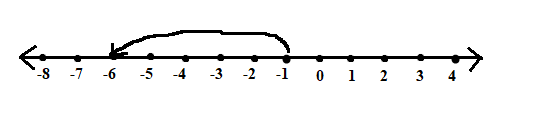EXERCISE 6.1

1) Write opposites of the following :
(a) Increase in weight.
Ans: Decrease in weight.
(b) 30 km north.
Ans: 30 km south.
(c) 326 BC.
(d) Loss of Rs.700.
Ans: Profit of Rs.700.
(e) 100 above sea level.
Ans: 100 below sea level.

2) Represent the following numbers as integers with appropriate signs.
(a) An aeroplane is flying at a height two thousand metre above
the ground.
Ans: + 2000.
(b) A submarine is moving at a depth, eight hundred metre below
the sea level.
Ans: – 800.
(c) A deposit of rupees two hundred.
Ans: + 200.
(d) Withdrawal of rupees seven hundred.
Ans: – 700.

3) Represent the following numbers on a number line :
(a) + 5
Ans: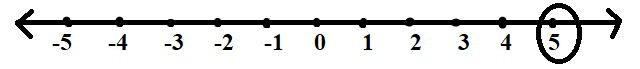(b) -10
Ans :(c) +8
Ans: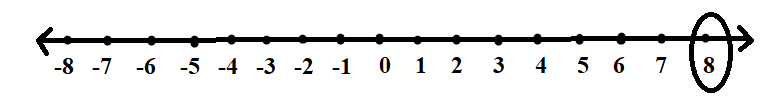(d) -1
Ans: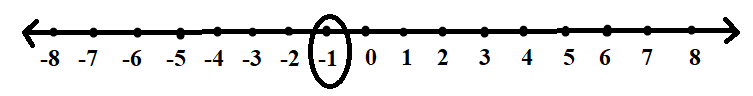(e) -6
Ans: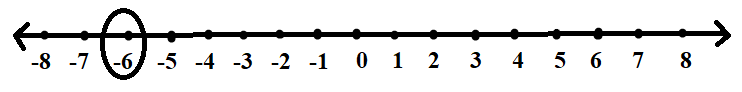4) Adjacent is a vertical number line, representing integers. Observe it and locate the following points.
(a) If point D is +8, then which point is −8?
Ans: F.
(b) Is point G a negative integers or a positive integer?
Ans: Negative integer.
(c) Write integers for points B and E.
Ans: B = +4 , E = −10.
(d) Which point marked on this number line has the least value?
Ans: E.
(e) Arrange all the points in decreasing order of value.
Ans: D, C, B, A, O, H, G, F, E.

5) Following is the list of temperature of five places in India, on a particular day of the year.

 Place Temperature Saichin 10˚ C below 0˚ Shimla 2˚ C below 0˚ Ahmedabad 30˚ C below 0˚ Delhi 20˚ C below 0˚ Srinagar 5˚ C below 0˚

(a) Write the temperature of these place in the form of integers in
the blank column.
Ans: -10˚ C, −2˚ C, +30˚ C, +20˚ C, −5˚ C.
(b) Following is the number line representing the temperature in
degree Celsius.
Ans: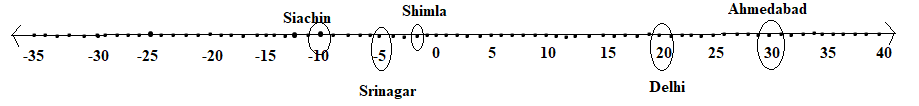(c) Plot the name of the city against its temperature.
Which is the coolest place?
Ans: Siachin.
(d) Write the name of the places whose temperature are above
10˚C .

6) In each of the following pairs, which number is to the right of the other on the number line?
(a) 2, 9               Ans: 9.

(b) −3, −8         Ans: −3.
(c) 0, −1             Ans: 0
(d) −11, 10         Ans: 10.
(e) −6, 6             Ans: 6
(f) 1, −100         Ans: 1.

7) Write all the integers between the given pairs(write them in the increasing order.)
(a) 0 and −7.
Ans: −6, −5,−4,−3,−2,−1.
(b) −4 and 4.
Ans: −3, −2, −1, 0, 1, 2, 3.
(c) −8 and −15.

Ans: −14, −13, −12, −11, −10, −9.
(d) −30 and −23.

Ans: −29, −28, −27, −26, −25, −24.

8)
(a) Write four negative integers greater than -20.

Ans: −10, −11, −12, −13.
(b) Write four negative integer less than -10.

Ans: −14, −15, −16, −17.

9) For the following statements write True (T) or False (F).If the statement is false, correct the statement.
(a) −8 is to the right of −10 on a number line.
Ans: True.
(b) −100 is to the right of −50 on a number line.
Ans: False, −100 is to the left of −50 on a number line.
(c) Smallest negative integer is −1.
Ans: False, greatest negative integer is −1.
(d) −26 is larger than −25.
Ans: False, −26 is smaller than −25.

10) Draw a number line and answer the following :
(a) Which number will we reach if we move 4 numbers to the right of −2.
Ans: +2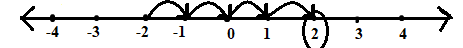(b) Which number will we reach if we move 5 numbers to the left of 1.
Ans: -4.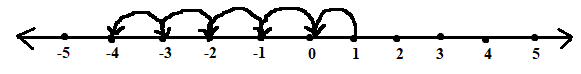(c) If we are at −8 on the number line, in which direction should we move to reach −13?
Ans: Since −13 lies to the left at −8, we should move towards left.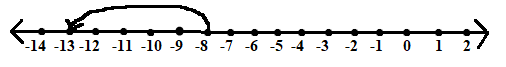(d) If we are at −6on the number line in which direction should we
move to reach −1?
Ans: Since −1 lies to right of −6 on the number line, we should move towards right direction.